### Home > INT2 > Chapter 12 > Lesson 12.1.3 > Problem12-39

12-39.
1. For each of the diagrams below, write and solve an equation for the given variable. For parts (a) and (b), assume that C is the center of the circle. Show all work. Homework Help ✎

2.  a.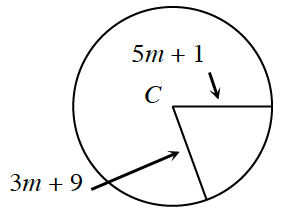b.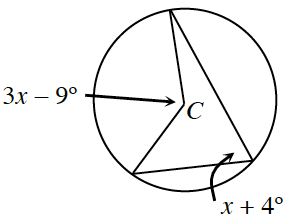c.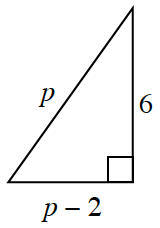d.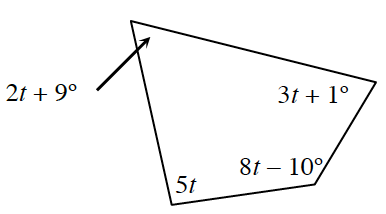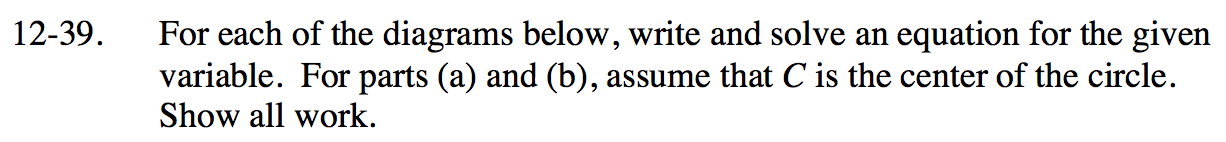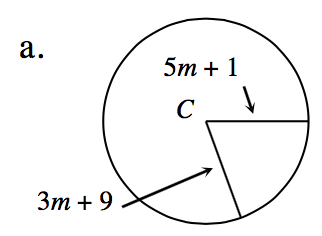Radii of circles always have the same distance.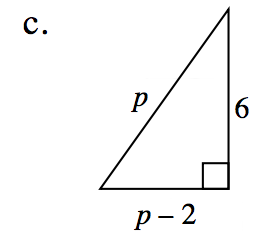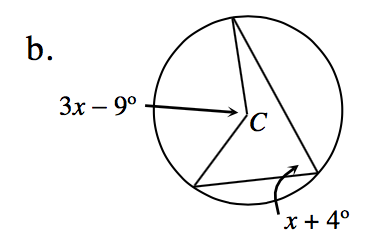Remember that an inscribed angle measures half of a central angle.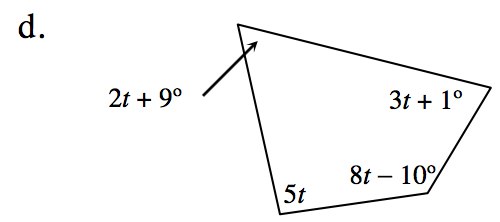The sum of a quadrilateral's interior angles is always 360°.